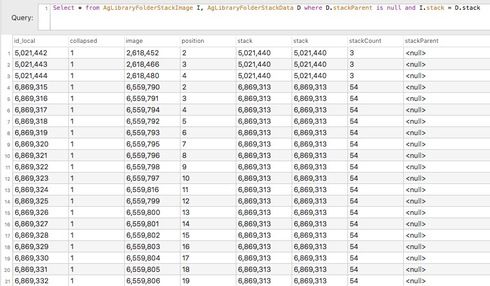##### kurt_hillig_gr9b9hw7oth354 Messages

•

132 Points

Wed, Mar 20, 2019 8:26 PM

# Lightroom Classic: Stacks with missing stackParents

See https://forums.adobe.com/message/10986625 for details.  It's not clear how it happened, but I've ended up with two stacks that can't be displayed, and images in them can't be found when searching by filename or keyword.

The problem with these two stacks is that neither one has a stack parent (the stackParent in the AgLibraryFolderStackData table is null) and neither stack shows an image in position 1 in the AgLibraryFolderStackImage table.  Here's what these two stacks look like in the database:I expect I'll have to edit the database by hand to fix this (I haven't tried yet, I need to make a backup copy of it first).  I'll post a follow-up once I've taken the plunge...

4 Messages

•

132 Points

3 y ago

As far as I can tell the manual update to the database did the trick!  All it took was to change the position of the last image in each stack to 1 in the AgLibraryFolderStackImage table (since each stack started at 2, no other impage positions were affected by this); a database trigger automatically set the stackParent to the correct value when this was done.

As I mentioned, I don't know how the database got into this state, but at least I know now how to fix things if it happens again...

4 Messages

•

132 Points

...and FYI, here's the SQL that fixed it:

List the data for stack 5021440, update it, and then show the result:

Select * from AgLibraryFolderStackImage I, AgLibraryFolderStackData D where I.stack = 5021440 and D.stack = I.stack

Update AgLibraryFolderStackImage set position = 1 where stack = 5021440 and image = 2618480

Select * from AgLibraryFolderStackImage I, AgLibraryFolderStackData D where I.stack = 5021440 and D.stack = I.stack

List the data for stack 6869313, update it, and then show the result:

Select * from AgLibraryFolderStackImage I, AgLibraryFolderStackData D where I.stack = 6869313 and D.stack = I.stack

Update AgLibraryFolderStackImage set position = 1 where stack = 6869313 and image = 6559846

Select * from AgLibraryFolderStackImage I, AgLibraryFolderStackData D where I.stack = 6869313 and D.stack = I.stack

Expand both stacks:

Update AgLibraryFolderStackImage set collapsed = 0 where stack = 5021440 or stack = 6869313